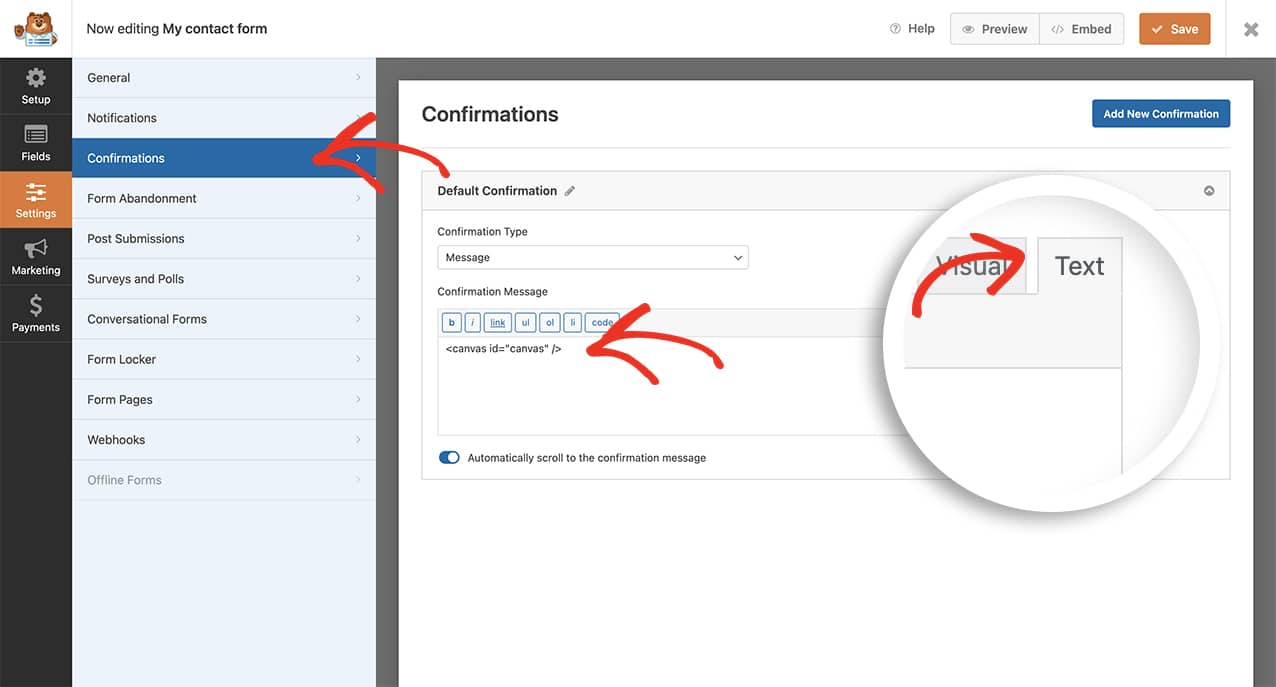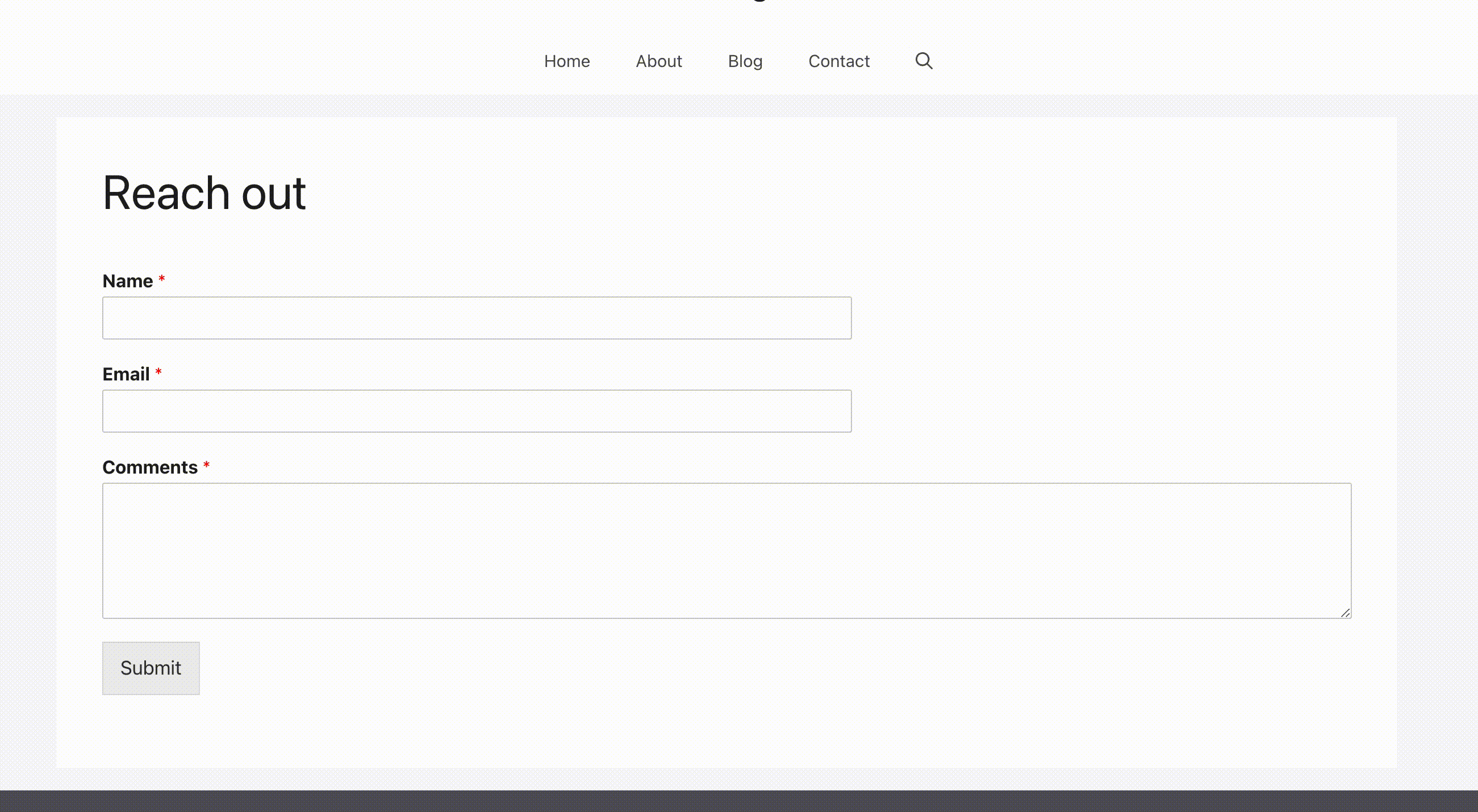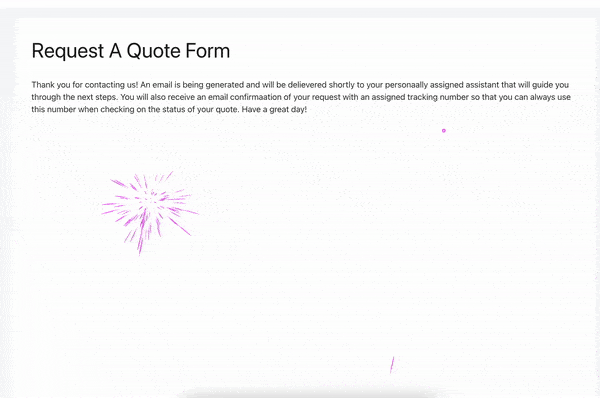# How to Add Confetti Animation to Confirmation Message

## Introduction

Would you like to add some cool confetti animation to your confirmation message? You can easily add some animation to the confirmation message that would have more of an interactive effect using CSS and JavaScript. In this tutorial, we’ll break down each step on how to achieve this.

First, you’ll need to create your form. For the purpose of this tutorial, we’ll just add a simple contact form with the Name, Email, and Paragraph Text form fields.

If you need any help in creating your form, please visit this documentation.

Once you’ve added your fields, click on the Settings tab and then click Confirmations. Inside the Confirmation Message window, we’re going to add an HTML canvas element that has an ID of canvas so that we can target exactly where the confetti will fall.

Once inside the Confirmations tab, remember to click the Text tab on the message box. You’ll need to do this in order to add pure HTML into this message area.Simply add `<canvas id="canvas" />`

Adding this canvas element and ID means that we can control where this confetti will appear. We only want it to show in the confirmation message so we’re adding an element that we can specifically target in our code snippet.

This snippet only runs on non-AJAX forms. You’d need to turn off your AJAX settings inside the form builder. To disable this setting go to the Settings tab inside the form builder and under the Advanced setting, disable the Enable AJAX form submission setting.## Adding the code snippet to create the confetti

Now it’s time to add the code snippet that will make the magic happen. For any assistance in adding a snippet to your site, please see this tutorial.

```/**
* Add confetti to the canvas element on the confirmation message
*
*/

function wpf_confetti_animation() {
?>

<script type="text/javascript">

//If the canvas ID does not exist on the page, this script will not run
if (document.querySelector( '#canvas' ) !== null) {

class Progress {
constructor(param = {}) {
this.timestamp        = null;
this.duration         = param.duration || Progress.CONST.DURATION;
this.progress         = 0;
this.delta            = 0;
this.progress         = 0;
this.isLoop           = !!param.isLoop;

this.reset();
}

static get CONST() {
return {
DURATION : 1000
};
}

reset() {
this.timestamp = null;
}

start(now) {
this.timestamp = now;
}

tick(now) {
if (this.timestamp) {
this.delta    = now - this.timestamp;
this.progress = Math.min(this.delta / this.duration, 1);

if (this.progress >= 1 && this.isLoop) {
this.start(now);
}

return this.progress;
} else {
return 0;
}
}
}

class Confetti {
constructor(param) {
this.parent         = param.elm || document.body;
this.canvas         = document.createElement("canvas");
this.ctx            = this.canvas.getContext("2d");
this.width          = param.width  || this.parent.offsetWidth;
this.height         = param.height || this.parent.offsetHeight;
this.length         = param.length || Confetti.CONST.PAPER_LENGTH;
this.yRange         = param.yRange || this.height * 2;
this.progress       = new Progress({
duration : param.duration,
isLoop   : true
});
this.rotationRange  = typeof param.rotationLength === "number" ? param.rotationRange
: 10;
this.speedRange     = typeof param.speedRange     === "number" ? param.speedRange
: 10;
this.sprites        = [];

this.canvas.style.cssText = [
"display: block",
"position: absolute",
"top: 0",
"left: 0",
"pointer-events: none"
].join(";");

this.render = this.render.bind(this);

this.build();

this.parent.appendChild(this.canvas);
this.progress.start(performance.now());

requestAnimationFrame(this.render);
}

static get CONST() {
return {
//CUSTOMIZE: This will adjust how wide the paper is
SPRITE_WIDTH  : 9,
//CUSTOMIZE: This will adjust how tall the paper is
SPRITE_HEIGHT : 16,
//CUSTOMIZE: This will adjust how much confetti appears, raise the number for less confetti
PAPER_LENGTH  : 100,
//CUSTOMIZE: This will control the rotation rate of each piece of confetti
ROTATION_RATE : 50,
//CUSTOMIZE: These are the default colors used for the confetti. You can change these numbers or add to them.
//CUSTOMIZE: Separate adding new colors by a comma after each new color added.
COLORS        : [
"#EF5350",
"#EC407A",
"#AB47BC",
"#7E57C2",
"#5C6BC0",
"#42A5F5",
"#29B6F6",
"#26C6DA",
"#26A69A",
"#66BB6A",
"#9CCC65",
"#D4E157",
"#FFEE58",
"#FFCA28",
"#FFA726",
"#FF7043",
"#8D6E63",
"#BDBDBD",
"#78909C"        ]
};
}

build() {
for (let i = 0; i < this.length; ++i) {
let canvas = document.createElement("canvas"),
ctx    = canvas.getContext("2d");

canvas.width  = Confetti.CONST.SPRITE_WIDTH;
canvas.height = Confetti.CONST.SPRITE_HEIGHT;

canvas.position = {
initX : Math.random() * this.width,
initY : -canvas.height - Math.random() * this.yRange
};

canvas.rotation = (this.rotationRange / 2) - Math.random() * this.rotationRange;
canvas.speed    = (this.speedRange / 2) + Math.random() * (this.speedRange / 2);

ctx.save();
ctx.fillStyle = Confetti.CONST.COLORS[(Math.random() * Confetti.CONST.COLORS.length) | 0];
ctx.fillRect(0, 0, canvas.width, canvas.height);
ctx.restore();

this.sprites.push(canvas);
}
}

render(now) {
let progress = this.progress.tick(now);

this.canvas.width  = this.width;
this.canvas.height = this.height;

for (let i = 0; i < this.length; ++i) {
this.ctx.save();
this.ctx.translate(
this.sprites[i].position.initX + this.sprites[i].rotation * Confetti.CONST.ROTATION_RATE * progress,
this.sprites[i].position.initY + progress * (this.height + this.yRange)
);
this.ctx.rotate(this.sprites[i].rotation);
this.ctx.drawImage(
this.sprites[i],
-Confetti.CONST.SPRITE_WIDTH * Math.abs(Math.sin(progress * Math.PI * 2 * this.sprites[i].speed)) / 2,
-Confetti.CONST.SPRITE_HEIGHT / 2,
Confetti.CONST.SPRITE_WIDTH * Math.abs(Math.sin(progress * Math.PI * 2 * this.sprites[i].speed)),
Confetti.CONST.SPRITE_HEIGHT
);
this.ctx.restore();
}

requestAnimationFrame(this.render);
}
}

(() => {
//CUSTOMIZE: This will control the speed of how fast the confetti falls, raise the number for a slower fall.
const DURATION = 8000,
//CUSTOMIZE: This number controls how much confetti will appear on the screen. For more confetti, raise the number.
LENGTH   = 120;

new Confetti({
width    : window.innerWidth,
height   : window.innerHeight,
length   : LENGTH,
duration : DURATION
});

setTimeout(() => {
new Confetti({
width    : window.innerWidth,
height   : window.innerHeight,
length   : LENGTH,
duration : DURATION
});
}, DURATION / 2);
})();

}
</script>
<?php
}
```

This code snippet can be customized to meet your needs if you’d like to change the colors of the confetti, the speed, the width etc. All you need to do to customize this is look in the comments section of the snippet above for CUSTOMIZE and a definition will show you what to customize.And that’s all you need! You’ve now successfully added a confetti animation to your confirmation message. Would you like to remove the default styling from the confirmation message? Check out our tutorial on How to Remove Confirmation Message Box Styling.

Action Reference: wp_footer

## Other Animation Effects

#### Firework Animation

The steps above are exactly the same but just use this snippet instead.

```/**
* Add firework to the canvas element on the confirmation message
*
*/

function wpf_fireworks_animation() {
?>

<script type="text/javascript">

// when animating on canvas, it is best to use requestAnimationFrame instead of setTimeout or setInterval
// not supported in all browsers though and sometimes needs a prefix, so we need a shim
window.requestAnimFrame = ( function() {
return window.requestAnimationFrame ||
window.webkitRequestAnimationFrame ||
window.mozRequestAnimationFrame ||
function( callback ) {
window.setTimeout( callback, 1000 / 60 );
};
})();

// now we will setup our basic variables for the demo
var canvas = document.getElementById( 'canvas' ),
ctx = canvas.getContext( '2d' ),
// full screen dimensions
cw = window.innerWidth,
ch = window.innerHeight,
// firework collection
fireworks = [],
// particle collection
particles = [],
// starting hue
hue = 120,
// when launching fireworks with a click, too many get launched at once without a limiter, one launch per 5 loop ticks
limiterTotal = 2,
limiterTick = 0,
// this will time the auto launches of fireworks, one launch per 80 loop ticks
timerTotal = 40,
timerTick = 0,
mousedown = false,
// mouse x coordinate,
mx,
// mouse y coordinate
my;

// set canvas dimensions
canvas.width = cw;
canvas.height = ch;

// now we are going to setup our function placeholders for the entire demo

// get a random number within a range
function random( min, max ) {
return Math.random() * ( max - min ) + min;
}

// calculate the distance between two points
function calculateDistance( p1x, p1y, p2x, p2y ) {
var xDistance = p1x - p2x,
yDistance = p1y - p2y;
return Math.sqrt( Math.pow( xDistance, 2 ) + Math.pow( yDistance, 2 ) );
}

// create firework
function Firework( sx, sy, tx, ty ) {
// actual coordinates
this.x = sx;
this.y = sy;
// starting coordinates
this.sx = sx;
this.sy = sy;
// target coordinates
this.tx = tx;
this.ty = ty;
// distance from starting point to target
this.distanceToTarget = calculateDistance( sx, sy, tx, ty );
this.distanceTraveled = 0;
// track the past coordinates of each firework to create a trail effect, increase the coordinate count to create more prominent trails
this.coordinates = [];
this.coordinateCount = 3;
// populate initial coordinate collection with the current coordinates
while( this.coordinateCount-- ) {
this.coordinates.push( [ this.x, this.y ] );
}
this.angle = Math.atan2( ty - sy, tx - sx );
this.speed = 2;
this.acceleration = 1.05;
this.brightness = random( 50, 70 );
}

// update firework
Firework.prototype.update = function( index ) {
// remove last item in coordinates array
this.coordinates.pop();
// add current coordinates to the start of the array
this.coordinates.unshift( [ this.x, this.y ] );

// cycle the circle target indicator radius
if( this.targetRadius < 8 ) {
} else {
}

// speed up the firework
this.speed *= this.acceleration;

// get the current velocities based on angle and speed
var vx = Math.cos( this.angle ) * this.speed,
vy = Math.sin( this.angle ) * this.speed;
// how far will the firework have traveled with velocities applied?
this.distanceTraveled = calculateDistance( this.sx, this.sy, this.x + vx, this.y + vy );

// if the distance traveled, including velocities, is greater than the initial distance to the target, then the target has been reached
if( this.distanceTraveled >= this.distanceToTarget ) {
createParticles( this.tx, this.ty );
// remove the firework, use the index passed into the update function to determine which to remove
fireworks.splice( index, 1 );
} else {
// target not reached, keep traveling
this.x += vx;
this.y += vy;
}
}

// draw firework
Firework.prototype.draw = function() {
ctx.beginPath();
// move to the last tracked coordinate in the set, then draw a line to the current x and y
ctx.moveTo( this.coordinates[ this.coordinates.length - 1][ 0 ], this.coordinates[ this.coordinates.length - 1][ 1 ] );
ctx.lineTo( this.x, this.y );
ctx.strokeStyle = 'hsl(' + hue + ', 100%, ' + this.brightness + '%)';
ctx.stroke();

ctx.beginPath();
// draw the target for this firework with a pulsing circle
ctx.arc( this.tx, this.ty, this.targetRadius, 0, Math.PI * 2 );
ctx.stroke();
}

// create particle
function Particle( x, y ) {
this.x = x;
this.y = y;
// track the past coordinates of each particle to create a trail effect, increase the coordinate count to create more prominent trails
this.coordinates = [];
this.coordinateCount = 5;
while( this.coordinateCount-- ) {
this.coordinates.push( [ this.x, this.y ] );
}
// set a random angle in all possible directions, in radians
this.angle = random( 0, Math.PI * 2 );
this.speed = random( 1, 10 );
// friction will slow the particle down
this.friction = 0.95;
// gravity will be applied and pull the particle down
this.gravity = 1;
// set the hue to a random number +-20 of the overall hue variable
this.hue = random( hue - 20, hue + 20 );
this.brightness = random( 50, 80 );
this.alpha = 1;
// set how fast the particle fades out
this.decay = random( 0.015, 0.03 );
}

// update particle
Particle.prototype.update = function( index ) {
// remove last item in coordinates array
this.coordinates.pop();
// add current coordinates to the start of the array
this.coordinates.unshift( [ this.x, this.y ] );
// slow down the particle
this.speed *= this.friction;
// apply velocity
this.x += Math.cos( this.angle ) * this.speed;
this.y += Math.sin( this.angle ) * this.speed + this.gravity;
this.alpha -= this.decay;

// remove the particle once the alpha is low enough, based on the passed in index
if( this.alpha <= this.decay ) {
particles.splice( index, 1 );
}
}

// draw particle
Particle.prototype.draw = function() {
ctx. beginPath();
// move to the last tracked coordinates in the set, then draw a line to the current x and y
ctx.moveTo( this.coordinates[ this.coordinates.length - 1 ][ 0 ], this.coordinates[ this.coordinates.length - 1 ][ 1 ] );
ctx.lineTo( this.x, this.y );
ctx.strokeStyle = 'hsla(' + this.hue + ', 100%, ' + this.brightness + '%, ' + this.alpha + ')';
ctx.stroke();
}

// create particle group/explosion
function createParticles( x, y ) {
// increase the particle count for a bigger explosion, beware of the canvas performance hit with the increased particles though
var particleCount = 80;
while( particleCount-- ) {
particles.push( new Particle( x, y ) );
}
}

// main demo loop
function loop() {
// this function will run endlessly with requestAnimationFrame
requestAnimFrame( loop );

// increase the hue to get different colored fireworks over time
hue += 0.5;

// normally, clearRect() would be used to clear the canvas
// we want to create a trailing effect though
// setting the composite operation to destination-out will allow us to clear the canvas at a specific opacity, rather than wiping it entirely
ctx.globalCompositeOperation = 'destination-out';
// decrease the alpha property to create more prominent trails
ctx.fillStyle = 'rgba(0, 0, 0, 0.5)';
ctx.fillRect( 0, 0, cw, ch );
// change the composite operation back to our main mode
// lighter creates bright highlight points as the fireworks and particles overlap each other
ctx.globalCompositeOperation = 'lighter';

// loop over each firework, draw it, update it
var i = fireworks.length;
while( i-- ) {
fireworks[ i ].draw();
fireworks[ i ].update( i );
}

// loop over each particle, draw it, update it
var i = particles.length;
while( i-- ) {
particles[ i ].draw();
particles[ i ].update( i );
}

// launch fireworks automatically to random coordinates, when the mouse isn't down
if( timerTick >= timerTotal ) {
if( !mousedown ) {
// start the firework at the bottom middle of the screen, then set the random target coordinates, the random y coordinates will be set within the range of the top half of the screen
fireworks.push( new Firework( cw / 2, ch, random( 0, cw ), random( 0, ch / 2 ) ) );
timerTick = 0;
}
} else {
timerTick++;
}

// limit the rate at which fireworks get launched when mouse is down
if( limiterTick >= limiterTotal ) {
if( mousedown ) {
// start the firework at the bottom middle of the screen, then set the current mouse coordinates as the target
fireworks.push( new Firework( cw / 2, ch, mx, my ) );
limiterTick = 0;
}
} else {
limiterTick++;
}
}

// mouse event bindings
// update the mouse coordinates on mousemove
canvas.addEventListener( 'mousemove', function( e ) {
mx = e.pageX - canvas.offsetLeft;
my = e.pageY - canvas.offsetTop;
});

// toggle mousedown state and prevent canvas from being selected
canvas.addEventListener( 'mousedown', function( e ) {
e.preventDefault();
mousedown = true;
});

canvas.addEventListener( 'mouseup', function( e ) {
e.preventDefault();
mousedown = false;
});